## ML Aggarwal Class 8 Solutions for ICSE Maths Model Question Paper 3

Questions 1 to 8 are of 1 mark each.
Question 1.
Product of rational number $$\frac{-4}{7}$$ and itsadditive inverse is
(a) $$\frac{16}{49}$$
(b) $$\frac{-16}{49}$$
(c) 1
(d) 0
Solution:
Product of rational number $$\frac{-4}{7}$$ and its
additive inverse – $$\left(\frac{-4}{7}\right)=\frac{4}{7}$$
= $$\frac{-4}{7} \times \frac{4}{7}=\frac{-16}{49}$$ (b)

Question 2.
What should be subtracted from $$\frac{-3}{5}$$ to get $$\frac{-2}{3}$$?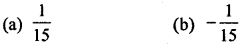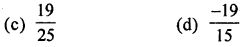Solution:Question 3.
The value of $$\left(\frac{2}{3}\right)^{0}+\left(\frac{2}{3}\right)^{-1}$$ isSolution: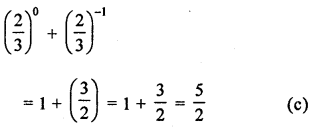Question 4.
Standard form of 0.00000000234 is
(a) 2.34 × 10-8
(b) 23.4 × 10-10
(c) 2.34 × 10-9
(d) 234 × 10-1
Solution:
0.00000000234 = 2.34 × 10-9 (c)

Question 5.
The volume of a cube is 216 m3. Length of its side is
(a) 3 m
(b) 6 m
(c) 9 m
(d) 12 m
Solution:
Volume of a cube = 216 m3
∴ Side – $$\sqrt{216}=\sqrt{6 \times 6 \times 6}$$ = 6 m (b)

Question 6.
When the difference of a 2-digit number ab (a > b) and the number obtained by reversing the digits is divided by 9, then quotient is
(a) a + b
(b) b – a
(c) a – b
(d) none of these
Solution:
The difference of the two-digit number ab (a > b)
and the number reversing the digits = $$\frac{a b-b a}{10}$$ = a – b (c)

Question 7.
Amar buys a toy for ₹ 120 and sells it for ₹ 144, his profit % is
(a) 5%
(b) 10%
(c) 20%
(d) 24%
Solution:
C.P. of a toy = ₹120
S.P. = ₹144
∴ Profit = S.P. – C.P. = ₹144 – ₹120 = ₹24
and Profit % = $$\frac{24 \times 100}{\text { C.P. }}=\frac{24 \times 100}{120}$$ = 20% (c)

Question 8.
If 567×8 is divisible by 9, then value of x is
(a) 1
(b) 2
(c) 3
(d) 4
Solution:
∵ 576×8 is divisible by 9
∴ Sum of digits 8 + x + 7 + 6 + 5 is also divisible by 9
⇒ 26 + x is divisible by 9
⇒ 26 + x = 27
⇒ x = 27 – 26 = 1
∴ x = 1

Section-B
Questions 9 to 14 are of 2 marks each.
Question 9.
Ramesh bought a fan for ₹ 1320 including 10% VAT. Find the price of the fan before VAT was added.
Solution:
Value of a fan including VAT = ₹1320
VAT = 10%
∴ Price of the fan before adding VATQuestion 10.Solution:A + 5 = 2 or 12
∴ A = 12 – 5 = 7

Question 11.
Find the value of x for which $$\left(-\frac{4}{5}\right)^{2} \times$$ $$\left(-\frac{5}{4}\right)^{2 x}=\frac{625}{256}$$.
Solution: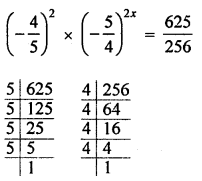Question 12.
Using appropriate properties of operations of rational numbers, evaluate: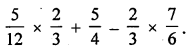Solution:Question 13.
What number should be subtracted from the sum of $$\frac{-5}{12}$$ and $$\frac{2}{3}$$ to get $$\frac{5}{4}$$ ?
Solution: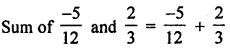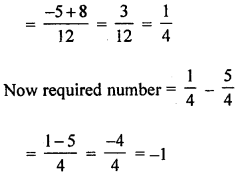Question 14.
If n(ξ) = 40, n(A) = 23, n(B’) = 28 and n(A ∪ B’) = 9, find n(A ∩ B).
Solution:
n(ξ) = 40, n(A) = 23, n(B’) = 28
n(A ∪ B’) = 9
n(B) – n(ξ) = -n(B’)
= 40 – 28 = 12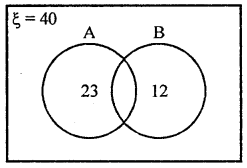n(A ∪ B) = n(ξ) – n(A ∪ B)’ = 40 – 9 = 31
∴ n(A ∩ B) = n(A) + n(B) – n(A ∪ B)
= 23 + 12 – 31 = 35 – 31 = 4

Section-C
Questions 15 to 24 are of 4 marks each.
Question 15.
In an auditorium, the number of rows is equal to the number of chairs in each row. If there are 5184 chairs in the auditorium, find the number of rows.
Solution:
In an auditorium number of rows = number of chairs in a row
Total chairs = 5184
Number of rows = $$\sqrt{5184}$$ = 72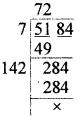Question 16.
Insert 5 rational numbers between $$\frac{1}{3}$$ and $$\frac{1}{2}$$.
Solution:
5 rational numbers between $$\frac{1}{3}$$ and $$\frac{1}{2}$$
LCM of 3, 2 = 6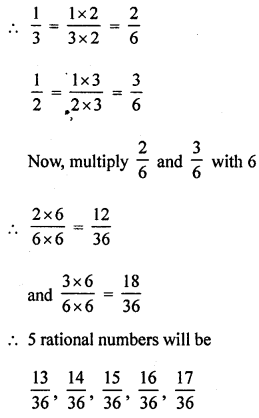Question 17.
Find the smallest number by which 10584 should be multiplied so that product is a perfect cube. Also find the cube root of the product.
Solution:
10584
Factors of 10584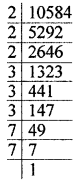= 2 × 2 × 2 × 3 × 3 × 3 × 7 × 7
Grouping the same factors in triplet, 7 × 7 are left unpaired.
∴ In order to complete a perfect cube, we shall multiply by 7.
So product = 10584 × 7 = 74088 and cube root = 2 × 3 × 7 = 42

Question 18.
In a 3-digit number unit’s digit is 2 less than hundred’s digit and hundred’s digit is 3 less than the ten’s digit. If the sum of the original number and number obtained by reversing the digits is 968, find the number.
Solution:
In a 3-digit number,
Let tens digit = x
Then hundreds, digit = x – 3
and unit digit = x – 3 – 2 = x – 5
∴ Number = (x – 5) + 10(x) + 100(x – 3)
= x – 5 + 10x + 100x – 300
= 111x – 305
By reversing the digits
Unit digit = x – 3
Ten’s digit = x
and hundred’s digit = x – 5
∴ Number = x – 3 + 10x + 100x – 500 = 111x – 503
According to the condition,
111x – 305 + 111x – 503 = 968
⇒ 222x – 808 = 968
222x = 968 + 808 = 1776
x = $$\frac{1776}{222}$$ = 8
and hundred’s digit = x – 5
∴ Number = 111 × 8 – 305 = 888 – 305 = 583

Question 19.
Rohit purchased some oranges at 3 for ₹5 and sold them at 5 for ₹12. Thus he gained ₹143 in all. How many oranges did he purchase?
Solution:
LCM of 3, 5 = 15
Let Rohit purchased = 15 oranges
∴ C.R of 15 oranges = $$\frac{15}{3}$$ × 5 = ₹25
and S.R of 15 oranges = $$\frac{15}{5}$$ × 12 = ₹36
Gain = S.P. – C.P. = ₹36 – ₹25 = ₹11
If gain is ₹11, then number apples = 15
and if gain is ₹143, then number of apples = $$\frac{15}{11}$$ × 143 = 195

Question 20.
How much per cent above the cost price should a shopkeeper mark his goods so that after allowing a discount of 20% he still earns a profit of 20%?
Solution:
Let cost price = ₹100
Profit = 20%∴ He should marked price ₹(150 – 100) = 50% above the cost price

Question 21.
Find the difference between S.I. and C.I. on ₹2000 for $$1 \frac{1}{2}$$ years at 6% p.a., compound interest being reckoned half-yearly.
Solution:
Principal (P) = ₹2000
Rate (R) = 6% p.a. or 3% half yearly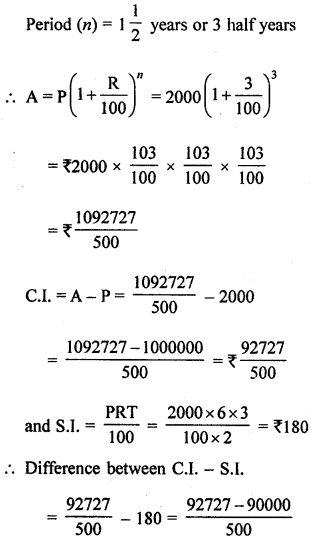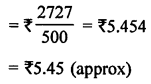Question 22.
If 5 men or 7 women can earn ₹903 in a day, how much will 10 men and 5 women earn in a day?
Solution:
5 men = 7 women
10 men = $$\frac{7 \times 10}{5}$$ = 14 women
10 men + 5 women = 14 + 5 = 19 women
7 women earn in day = ₹903
7 women : 19 women :: ₹903 : x (More women, more earning)
∴ 7 × x = 19 × 903
x = $$\frac{19 \times 903}{7}$$ = ₹2451
Hence 10 men and 5 women will earn ₹2451

Question 23.
A hostel had rations for 150 students for 60 days. After 12 days, 30 more students join the hostel. How long will the remaining rations last?
Solution:
There is rations in a hostel for 150 students for 60 days.
After 12 days, 30 more students join
∴ Now total students = 150 + 30 = 180
Remaining days = 60 – 12 = 48
150 students : 180 students :: 48 days : x
More students, less days
∴ By inverse variation
180: 150 :: 48 : x
x = $$\frac{150 \times 48}{180}$$ = 40 days
∴ Remaining ration will last for 40 days.

Question 24.
Simplify the following expression and write in the exponential form:
3-5 × 32 ÷ 36 × (22 × 3)2 + $$\left(\frac{2}{3}\right)^{-1}$$ + 2-1 + $$\left(\frac{1}{19}\right)^{-1}$$.
Solution:
3-5 × 32 ÷ 36 × (22 × 3)2 + $$\left(\frac{2}{3}\right)^{-1}$$ + 2-1 + $$\left(\frac{1}{19}\right)^{-1}$$.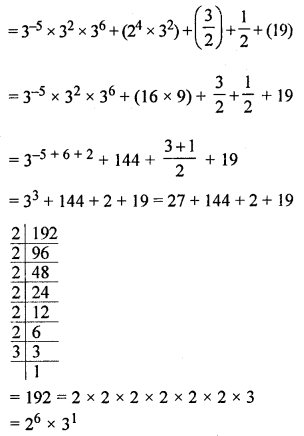Section-D
Questions 25 to 29 are of 6 marks each.
Question 25.
Ramesh scored 30% marks in an exam and failed by 20 marks, while Suresh scored 40% marks and got 40 marks more than the minimum marks required to pass the exam. Find the maximum marks and pass percentage.
Solution:
Ramesh scored 30% marks but failed by 20 marks
Suresh scored 40% marks and got 40 marks more than pass marks
∴ Difference in % = 40 – 30 = 10%
But actual difference = 20 + 40 = 60
∴ 10% of total marks = 60
∴ Total marks = $$\frac{60 \times 100}{10}$$ = 600
and pass marks = 30% of 600 + 20
= $$\frac{600 \times 30}{100}$$ + 20 = 180 + 20 = 200
and percentage = $$\frac{200}{600}$$ × 100
= $$\frac{100}{3} \%=33 \frac{1}{3} \%$$

Question 26.
If ξ = {x | x ϵ W, x < 20}, A = {factors of 18} and B = {multiples of 3}, find
(i) A ∪ B
(ii) A ∩ B
(iii) (A ∪ B)’.
Also verify that n(A ∪ B) = n(A) + n(B) – n(A ∩ B).
Solution:
ξ = (x | x ϵ W, x < 20} = {0, 1, 2, 3, 4, ………, 19}
A = (factors of 18} = (1,2, 3, 6, 9, 18}
n(A) = 6
B = (multiples of 3} = {3, 6, 9, 12, 15, 18}
n(B) = 6
(i) A ∪ B = {1, 2, 3, 6, 9, 18} ∪ (3, 6, 9, 12, 15, 18}
= {1,2, 3, 6, 9, 18, 12, 15}
⇒ n(A ∪ B) = 8
(ii) A ∩ B = {1, 2, 3, 6, 9, 18} ∩ (3, 6, 9, 12, 15, 18}
= {3, 6, 9, 18}
⇒ n(A ∩ B) = 4
(iii) (A ∪ B)’ = ξ – (A ∪ B)
= {4, 5, 7, 10, 11, 13, 14, 16, 17, 19}
n(A ∪ B) = n(A) + n(B) – n(A ∩ B)
LHS = n(A ∪ B) = 8
RHS = n(A) + n(B) – n(A ∩ B)
= 6 + 6 – 4 = 12 – 4 = 8
Hence LHS = RHS

Question 27.
A and B together can do a piece of work in 15 days; B and C together can do it in 12 days; C and A can do it in 20 days. How long will they take to finish the work, working together? Also find the number of days taken by each to do the same work, working alone.
Solution:
A and B’s 1 day’s work = $$\frac{1}{15}$$ ….(i)
B and C’s 1 day’s work = $$\frac{1}{12}$$ ….(ii)
C and A’s 1 day’s work = $$\frac{1}{20}$$ ….(iii)
2(A + B + C)’s 1 day’s work∴ (A + B + C)’s 1 day’s work = $$\frac{1}{5} \times \frac{1}{2}=\frac{1}{10}$$
∴ They all together will finish the work in 10 days
A’s 1 day’s work = $$\frac{1}{10}-\frac{1}{12}$$
[∵ Work finished by all together – B and C’s 1 day’s work]
= $$\frac{6-5}{60}=\frac{1}{60}$$
∴ He will finish the work in 60 days
B’s 1 day’s work = $$\frac{1}{10}-\frac{1}{20}$$
[∵ Work finished by all together – C and A’s 1 day’s work]
= $$\frac{2-1}{20}=\frac{1}{20}$$
∴ He will finish the work in 20 days
C’s 1 day’s work = $$\frac{1}{10}-\frac{1}{15}$$
[∵ Work finished by all together – A and B’s 1 day’s work]
= $$\frac{3-2}{30}=\frac{1}{30}$$
∴ He will finish the work in 30 days.

Question 28.
Is 31104 a perfect square number? If not then find the smallest number by which 31104 should be divided so that quotient is a perfect square. Also, find the square root of the quotient.
Solution:
31104
Factorising, we get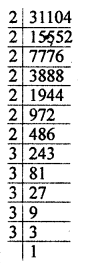31104 = 2 × 2 × 2 × 2 × 2 × 2 × 2 × 3 × 3 × 3 × 3 × 3
Pairing the same kind of factors,
we find that 2 × 3 are left without pairing.
So, 2 × 3 i.e. 6 is the least number by which 31104 is to be
divided for getting a perfect square.
Hence the number will be = 31104 + 6
= 5184
∴ Square root = 2 × 2 × 2 × 3 × 3 = 72

Question 29.
Three numbers are in the ratio 1:3:5 and sum of their cubes is 52479. Find the numbers.
Solution:
Ratio in 3 numbers =1 : 3 : 5
Let first number = x
Second number = 3x
Third number = 5x
According to the condition,
(x)3 + (3x)3 + (5x)3 = 52479
⇒ x3 + 27x3 + 125x3 = 52479
⇒ 153x3 = 52479
⇒ x3 = $$\frac{52479}{153}$$ = 343
x3 = 7 × 7 × 7 = 73
∴ x = 7
∴ First number = x = 7
Second number = 3x = 3 × 7 = 21
Third number = 5x = 5 × 7 = 35
Hence numbers are 7, 21, 35